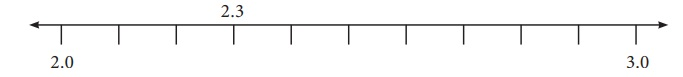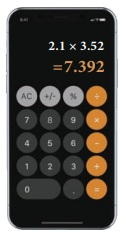Home | | Maths 7th Std | Rounding of Decimals

# Rounding of Decimals

Rounding of decimals would be really useful for estimating amounts of money, duration of time, measure of distances and many other physical quantities.

Rounding of Decimals

Rounding of decimals would be really useful for estimating amounts of money, duration of time, measure of distances and many other physical quantities. Let us learn the same here.

MATHEMATICS ALIVE - Decimals in real lifeYou can round decimals just in the same way as you round whole numbers.

To round a decimal :

1. First underline the digit that is to be rounded. Then look at the digit to the right of the underlined digit.

2. If that digit is less than 5, then the underlined digit remains the same.

3. If that digit is greater than or equal to 5, add 1 to the underlined digit.

4. After rounding of, ignore all the digits after the underlined digit.

Example 1.1

Round 2.367 to the nearest whole number.

Solution

Underline the digit to be rounded - 2.367

Since the digit next to the underlined digit is 3 which is less than 5, the underlined digit 2 remains the same.

Also look at the number line shown below :On the number line, we observe that 2.3 is closer to 2.0 than 3.0

Hence, the rounded value of 2.367 to the nearest whole number is 2.

Example 1.2

Round 99.95 to the nearest tenth place.

Solution

Underline the digit to be rounded 99.95.

Since the digit right to the tenth place is 5, we add 1 to the tenth place (underlined digit) of 99.95 and we get 100.0.

Look at the number line shown below:So, the rounded value of 99.95 is 100.0.

Example 1.3

Round 52.6583 upto 2 places of decimal.

Solution

Round 52.6583 upto 2 places of decimal means round to the nearest hundredths place.

Underline the digit in the hundredth place of 52.6583 gives 52.6583.

We observe that the digit after the hundredth place value is 8 which is more than 5.

Therefore, we should add 1 to the underlined digit. Hence, we get 52.66.

So the rounded value of 52.6583 upto 2 places of decimal is 52.66.

Example 1.4

Round 189.0007 upto 3 places of decimal.

Solution

Round 189.0007 upto 3 places of decimal means rounding to the nearest thousandth place

Underline the digit in the thousandth place of 189.0007 gives 189.0007

In 189.0007 we observe that the digit next to the thousandth place value is 7, which is greater than 5.

Therefore, we should add 1 to the underlined digit. Hence, we get 189.001.

So the rounded value of 189.0007 upto 3 places of decimal is 189.001

Now a days calculators, computers and even mobile phones were used for multiplication and division of decimals. But, actually multiplication of decimals using logarithm tables will be more useful and handy.Tags : Number System | Term 3 Chapter 1 | 7th Maths , 7th Maths : Term 3 Unit 1 : Number System
Study Material, Lecturing Notes, Assignment, Reference, Wiki description explanation, brief detail
7th Maths : Term 3 Unit 1 : Number System : Rounding of Decimals | Number System | Term 3 Chapter 1 | 7th Maths